College Physics : Harmonic Motion

Example Questions

Example Question #34 : College Physics

A pendulum is made up of a small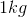mass that hangs on the end of a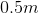long string of negligible mass. The pendulum is displaced by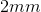and allowed to undergo harmonic motion. What is the angular frequency of the resulting motion?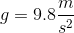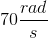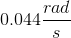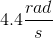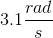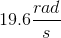Explanation:

The angular frequency of a simple pendulum is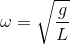, whereis the length of the pendulum.

Example Question #35 : College Physics

Which of the following is not an example of simple harmonic motion?

The mass on a pendulum moving back and forth

A child swinging on a swing set

A book falling to the ground

A plucked string vibrating on a guitar

A book falling to the ground

Explanation:

For this question, we need to recall what simple harmonic motion is. Remember that it is a periodic motion where the restoring force depends on the displacement of the object undergoing these motions. So to answer this question, we need to keep this idea in mind and see which example doesn't match up.

A mass on a pendulum moving back and forth is clearly an example of simple harmonic motion. As the mass moves further from the center in either direction, it experiences a greater and greater force in the opposite direction.

A child swinging on a swing set is another correct example. This situation is analogous to the mass on a pendulum swinging back and forth.

A vibrating guitar string is yet another example of simple harmonic motion. After it is plucked, the string oscillates back and forth.

Finally, a book falling to the ground does not represent harmonic motion. Once the book is released from rest, we intuitively know that it will fall to the ground and will then stay there; in no way is there any periodic motion.

Example Question #1 : Harmonic Motion

If the mass of a simple pendulum is quadrupled, then its period __________.

remains the same

is reduce to one quarter

doubles

remains the same

Explanation:

We know that the equation for the period of a simple pendulum is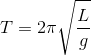. This equation does not depend on mass. It is only affected by the length of the pendulum (L) and the gravitational constant (g). Therefore, adding mass to the pendulum will not effect the period, so the period remains the same.

Example Question #37 : College Physics

A violin string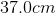long has a linear density of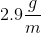. What is the string tension if the second harmonic has a frequency of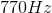?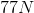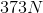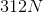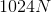Explanation:

Since we are solving for string tension, we need to use the frequency equation with the tension variable in it. That equation is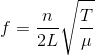whereis the frequency,is the number of the harmonic,is the length of the string,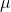is the linear density of the string, and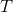is the tension of the string.

We are given: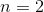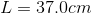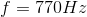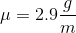Next we must convert the length ofto meters which is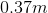and the mass density ofto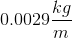. Then we plug in our known values into the equation and solve for the string tension. The result is.

Example Question #1 : Harmonic Motion

For a simple harmonic motion governed by Hooke's Law,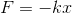, ifis the period then the quantity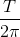is equivalent to which of the following?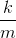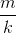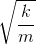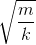Explanation:

We know that T is the period. The equation for T is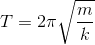for harmonic motion.

Solve forby dividing the equation byon both sides. The result is, which is the answer.

Example Question #2 : Harmonic Motion

A pendulum on earth has a period of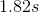. What is it's period on Mars with it's gravity is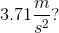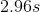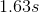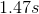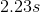Explanation:

First, we need to find the length of the pendulum. Begin with the equation for finding the period of a pendulum:solve forto get: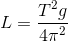Now we can plug in our given values: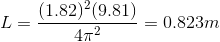Since we have the length of the pendulum determined, we can now find the period of the pendulum on Mars: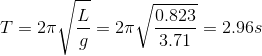All College Physics Resources